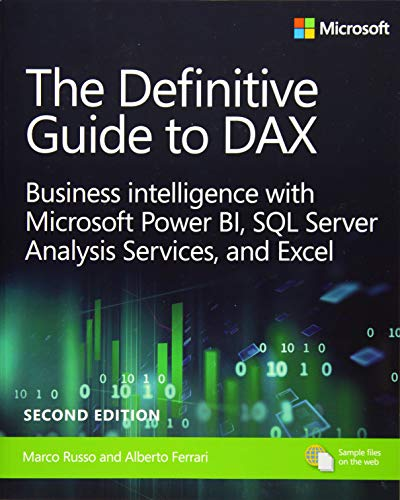# The Definitive Guide to DAX, 2nd Edition

Rating: 4.6 out of 5 64 reviews
• Length: 768 pages
• Edition: 2
• Publisher:
• Publication Date: 2019-07-02
• ISBN-10: 1509306978
• ISBN-13: 9781509306978

## Book Description

Now expanded and updated with modern best practices, this is the most complete guide to Microsoft’s DAX language for business intelligence, data modeling, and analytics. Expert Microsoft BI consultants Marco Russo and Alberto Ferrari help you master everything from table functions through advanced code and model optimization. You’ll learn exactly what happens under the hood when you run a DAX expression, and use this knowledge to write fast, robust code. This edition focuses on examples you can build and run with the free Power BI Desktop, and helps you make the most of the powerful syntax of variables (VAR) in Power BI, Excel, or Analysis Services. Want to leverage all of DAX’s remarkable capabilities? This no-compromise “deep dive” is exactly what you need.

Perform powerful data analysis with DAX for Power BI, SQL Server, and Excel

• Master core DAX concepts, including calculated columns, measures, and calculation groups
• Work efficiently with basic and advanced table functions
• Understand evaluation contexts and the CALCULATE and CALCULATETABLE functions
• Perform time-based calculations
• Use calculation groups and calculation items
• Use syntax of variables (VAR) to write more readable, maintainable code
• Express diverse and unusual relationships with DAX, including many-to-many relationships and bidirectional filters
• Master advanced optimization techniques, and improve performance in aggregations
• Optimize data models to achieve better compression
• Measure DAX query performance with DAX Studio and learn how to optimize your DAX

``````Chapter 1. What is DAX?
Chapter 2. Introducing DAX
Chapter 3. Using basic table functions
Chapter 4. Understanding evaluation contexts
Chapter 5. Understanding CALCULATE and CALCULATETABLE
Chapter 6. Variables
Chapter 7. Working with iterators and with CALCULATE
Chapter 8. Time intelligence calculations
Chapter 9. Calculation groups
Chapter 10. Working with the filter context
Chapter 11. Handling hierarchies
Chapter 12. Working with tables
Chapter 13. Authoring queries
Chapter 16. Advanced calculations in DAX
Chapter 17. The DAX engines
Chapter 18. Optimizing VertiPaq
Chapter 19. Analyzing DAX query plans
Chapter 20. Optimizing DAX``````